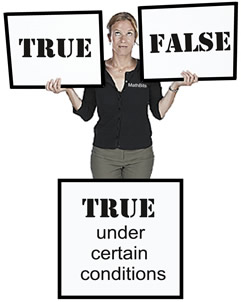Truth Values of Equations MathBitsNotebook.com Terms of Use   Contact Person: Donna RobertsWhen you solve an equation, you usually get an answer that looks like x = 10, where you have a variable equal to some value. This is the desired type of answer. But there are two other "strange" options that may appear when you solve an equation. Let's take a look.

 Most equations are TRUE when certain values are substituted for the variable (such as x), and are FALSE for ALL other values. A value that makes an equation TRUE is called a "solution". There are, however, "unique" equations that are always TRUE or always FALSE, no matter what values are substituted. So, we have three possible situations with linear equations: Equations TRUE for a certain value. (almost always the case) Equations TRUE for ALL values. (may happen) Equations FALSE for ALL values. (may happen)Equations that are TRUE under certain conditions:
These are the majority of algebraic equations.
Consider x - 1 = 9.
This equation has one solution (that makes the equation TRUE) when x = 10, because 10 - 1 = 9 is true. For all other values of x, the equation is FALSE. Such equations can be referred to as conditional equations because they are TRUE only under certain conditions. For all other values, these equations will be FALSE.Remember: When an equation is a linear equation (of degree one), it has only one value as its solution. The only exceptions are the "strange" situations mentioned in the next two bullets.Equations that are ALWAYS TRUE:
Consider x + 7 = 7 + x.
This equation has an infinite number of solutions. Any value you choose for x will make the equation a TRUE statement. This type of equation is called an identity, and the solution set is all real numbers.

When you solve this type of equation, the variable will disappear, and you will be left with a TRUE statement about some numeric value. In this example, the "x" disappears and you are left with the statement, 7 = 7.

A few other examples:
 4(x - 1) = 4x - 4 x + x = 2x (x + 3)(x - 3) = x2 - 9 Equations that are identities tend to be statements involving "properties" or "rules", such as a property of the real numbers (commutative property, distributive property, etc.), an arithmetic operation on the variable (addition, subtraction, etc), a rule for factoring, and so on. Both sides of the equation represent the same algebraic expression, just written in a different manner.If you solve an equation and you end up with an obvious number identity (some number equal to itself), such as 5 = 5, you'll know that the original equation is also an identity, with an infinite number of solutions.Equations that are ALWAYS FALSE:
Consider x + 7 = x.
This equation has no solutions. No matter what value you choose for x, the equation will be a FALSE statement. Such statements can be referred to as contradictions.
The term "contradiction" refers to statements that are opposed to one another. In relation to equations, it implies that the two sides of the equation are not in agreement, thus no solution will be found.

When you solve this type of equation, the variable will disappear, and you will be left with a FALSE numerical statement. In this example, the "x" disappears and you are left with the statement 7 = 0.If you solve an equation and you end up with a numerical contradiction, such as 1 = 2, you'll know that the original equation is also a contradiction and has no solutions.